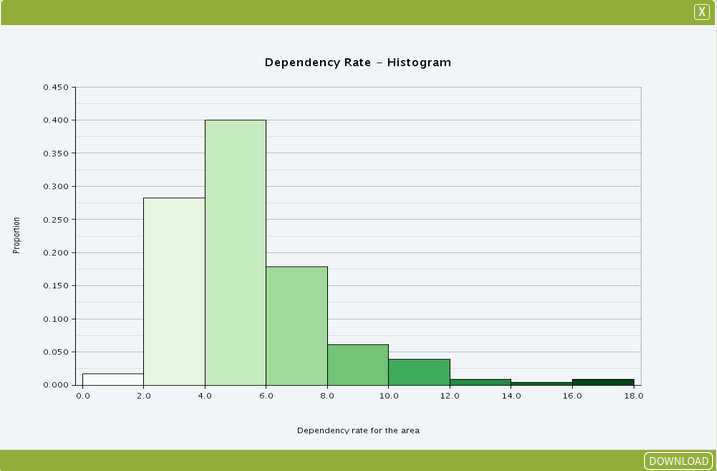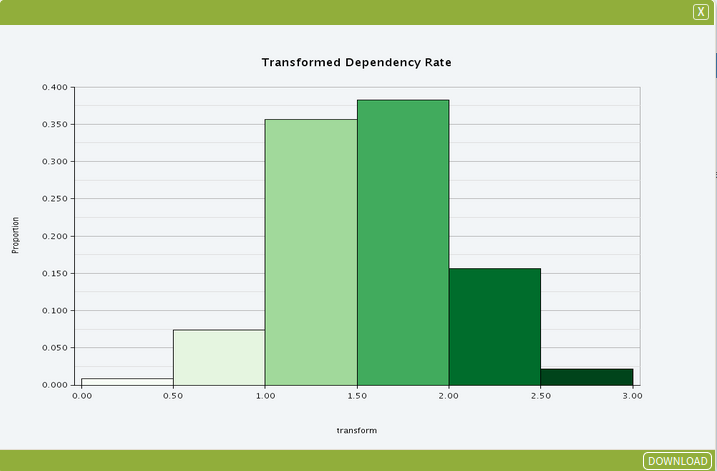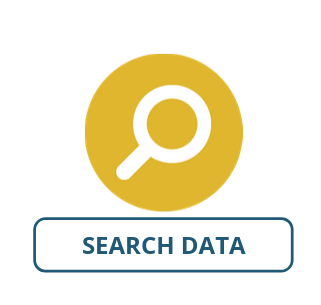# Generate Transformation

The Generate Transformation tool allows you to perform mathematical operations on an aggregated dataset. You will be able to download the data as a csv or json file. Should you require to download the datset in form of a shapefile (shp), please use the Spatialise Aggregated Dataset tool in the Tools pane, or the Generate Transformation (Spatial) tool if running on a non-aggregated dataset.

### Set Up

In this example, we will explore the distribution of the dependency rate of the population across SA2s in Brisbane in 2016.

• Select Greater Brisbane (gccsa_2016/3GBRI) as your area
• Select NATSEM – Social and Economic Indicators – Dependency Rate SA2 2016 as your dataset with the following variables:
• SA2 Code 2016
• SA2 Name 2016
• Dependency rate for the area

After adding the data, follow the instructions of the Histogram tool user guide to create a chart for the Dependency rate for the area variable.

Once you have completed these steps, open the image in the Visualise pane to explore the distribution of the dependency rate. Your example should look similar to this and show a positively skewed distribution. If you are planning to perform further analysis on the data, you will need to correct the skew by using a mathematical function – in this case a logarithm transformation.You are now ready to use the Generate Transformation tool – follow the inputs instructions below to see how to do this.

### Inputs

To perform the transformation, open the Generate Transformation tool (Tools → Data Processing → Generate Transformation) and enter the parameters as shown in the image below (each of the parameters is explained in more detail below the image).• Dataset Input: This is the dataset that contains the columns you would like to include in the calculation. In this example, we will use the dataset NATSEM – Social and Economic Indicators – Dependency Rate SA2 2016
• Operand 1: This represents the ‘left-hand side’ of the equation. In this instance, we will use the Dependency rate for the area
• Operator: This represents the mathematical function that you would like to use to create the new column. In this instance, we are using the logarithm function (log) to correct the right-hand skew presented in the histogram above and even out the population distribution per area.
• Other operators include:
• log2 – Base 2 logarithm transformation – Strong transformation which reduces right skewness, does not work on negative values
• log10 – Base 10 logarithm transformation – Strong transformation which reduces right skewness, does not work on zero or negative values
• exp – Exponential or natural log transformation – Strong transformation which reduces right skewness, does not work on zero or negative values
• x^2 – Square transformation – Moderate transformation which reduces left skewness, does not work on negative values.
• sqrt – Square root transformation – Moderate transformation which reduces right skewness, does not work on negative values
• 1/x – Repciprocal transformation – Very strong transformation, alters the interpretation of the data, does not work on zero values
• New Column Name: This will be the new column in the output table. It is important that you only include letters, numbers and underscores (no spaces or other characters!) in this column. Also, it can only start with a letter – no number at the start!

Once you have entered your parameters, click Run Tool.

### Outputs

Once your tool as run, a new dataset will appear in your Data panel named Output: GenerateTransformation XXX. It might be a good idea to rename it at this point. If you open the dataset up, you will see the new column that you have created at the right-hand side of the table.In our example here, the log function in the Generate Transformation tool was used to reduce the skew. You can verify this by repeating the steps above and creating another Histogram of the data:You can now map this output dataset and column as you normally would using the Choropleth visualisation function.

### Looking for Spatial Data?

You can browse the AURIN Data Discovery:### How can you Create Impact?